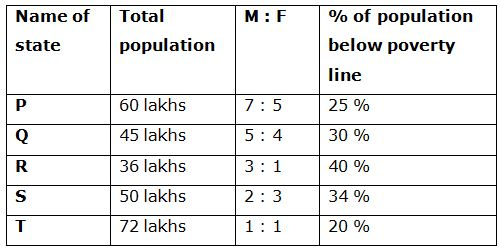# IBPS RRB PO Prelims Quantitative Aptitude Questions 2019 (Day-12)

Dear Aspirants, Our IBPS Guide team is providing new series of Quantitative Aptitude Questions for IBPS RRB PO Prelims 2019 so the aspirants can practice it on a daily basis. These questions are framed by our skilled experts after understanding your needs thoroughly. Aspirants can practice these new series questions daily to familiarize with the exact exam pattern and make your preparation effective.

Check here for IBPS RRB Prelims Mock Test 2019

### Click Here to Subscribe Crack High Level Puzzles & Seating Arrangement Questions PDF 2019 Plan

[WpProQuiz 6738]

Directions (Q. 1 – 5): What approximate value should come in place of question mark (?) in the following questions?

1) (32/7) of [8955.21 – 2368.13] + 234.5 =? + 3429.87

a) 31560

b) 22440

c) 26920

d) 34280

e) 17650

2) 24 % of 849 + 56 % of 1299 – 456.23 ÷ 4 =? + (8.11)2

a) 686

b) 612

c) 575

d) 438

e) 754

3) 17 % of ? – 2054 ÷ 12.78 – 222 ÷ 3.01 = 57

a) 1700

b) 2000

c) 2200

d) 1300

e) 2500

4) 5 (¼) + 3 (5/8) – 4 (2/3) + 2 (½) =? – 4 (3/4)

a) 20

b) 6

c) 24

d) 12

e) 30

5) [(46.04)2 ÷ 23 × 6.11] ÷ 12 = 2.99 ×? – 788 ÷ 3

a) 158

b) 103

c) 215

d) 267

e) 99

Directions (Q. 6 – 10): Study the following information carefully and answer the given questions:

The following table shows the total population in 5 different states, the ratio of male and female among them and the percentage of population below poverty line.6) Find the difference between the total male population in state P and R together to that of total female population in state Q and T together?

a) 15 lakhs

b) 22 lakhs

c) 6 lakhs

d) 10 lakhs

e) None of these

7) Find the ratio between the above poverty line population in state R to that of the below poverty line population in state S?

a) 108: 85

b) 75: 62

c) 52: 43

d) 11: 5

e) None of these

8) Find the total number of female population in state P, R and S together

a) 72 lakhs

b) 90 lakhs

c) 56 lakhs

d) 64 lakhs

e) None of these

9) The above poverty line population in state P and Q together is approximately what percentage of total population in state S?

a) 132 %

b) 153 %

c) 120 %

d) 105 %

e) 94 %

10) The below poverty line population in state R and T together is what percentage more/less than the total male population in state Q and S together?

a) 36 % more

b) 15 % less

c) 36 % less

d) 4 % more

e) 15 % more

Direction (1-5) :

(32/7) of [8955 – 2368] + 235 = x + 3430

(32/7)*(6587) + 235 – 3430 = x

X = 30112 + 235 – 3430 = 26917

X = 26920

24 % of 850 + 56 % of 1300 – 456 ÷ 4 = x + 82

(24/100)*850 + (56/100)*1300 – (456/4) – 64 = x

204 + 728 – 114 – 64 = x

X = 754

(17/100)*x – (2054/13) – (222/3) = 57

(17/100)*x = 57 + 158 + 74

(17/100)*x = 289

X = 1700

5 (¼) + 3 (5/8) – 4 (2/3) + 2 (½) + 4 (¾) = x

X = 5 + 4 – 5 + 3 + 5 = 12

[(46)2 ÷ 23 × 6] ÷ 12 = 3x – 789 ÷ 3

(46*46*6)/(23*12) = 3x – (789/3)

46 + 263 = 3x

3x = 309

X = 103

Direction (6-10) :

The total male population in state P and R together

= > 60*(7/12) + 36*(3/4)

= > 35 + 27 = 62 lakhs

The total female population in state Q and T together

= > 45*(4/9) + 72*(1/2)

= > 20 + 36 = 56 lakhs

Required difference = 62 – 56 = 6 lakhs

The above poverty line population in state R

= > 36*(60/100)

The below poverty line population in state S

= > 50*(34/100)

Required ratio = [36*(60/100)]: [50*(34/100)]

= > 108: 85

The total number of female population in state P, R and S together

= > 60*(5/12) + 36*(1/4) + 50*(3/5)

= > 25 + 9 + 30 = 64 lakhs

The above poverty line population in state P and Q together

= > 60*(75/100) + 45*(70/100)

= > 45 + 31.5 = 76.5 lakhs

Total population in state S = 50 lakhs

Required % = (76.5/50)*100 = 153 %

The below poverty line population in state R and T together

= > 36*(40/100) + 72*(20/100)

= > 14.4 + 14.4 = 28.8 lakhs

The total male population in state Q and S together

= > 45*(5/9) + 50*(2/5)

= > 25 + 20 = 45 lakhs

Required % = [(45 – 28.8)/45]*100 = 36 % less

5 1 vote
Rating